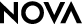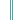World in the BalanceStudent Handout

Calculating Population Growth

Use the instructions on this handout to calculate population growth estimates for your assigned countries.

Procedure

1. Multiply the initial population by the growth rate. This will give you the number of individuals that are added to the population in a 10-year period. (This number should be rounded up, since partial individuals do not exist in the real world.)

2. Add the result from Step 1 to the initial population to get the new population after 10 years.

3. For the next 10-year period, the new population size becomes the starting population value. Multiply the new population size by the growth rate. As before, add the resulting number of individuals to the starting population to calculate the new population size after 20 years.

4. Repeat the process until each country's population has doubled. Note that because you are looking a 10-year periods, the population may not be exactly double in size at the end of a period. For instance, in the example given, you would stop after 30 years, when the population reaches 124.

Sample Calculation
Here is a sample calculation for a country with a 10-year growth rate of .25. The country's population doubles soon after 20 years.

 Starting Population 10-year Growth Rate Number ofNew Individuals New Population Size Initial 50 .25 12.5 (13) 63 After 10 years 63 .25 15.75 (16) 79 After 20 years 79 .25 19.75 (20) 99 After 30 years 99 .25 24.75 (25) 124

-->